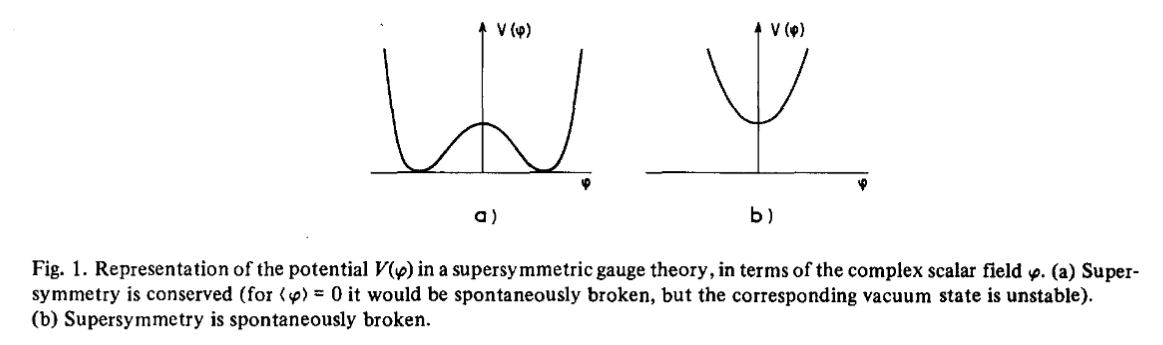Contents

supersymmetry

# Contents

## Idea

The spontaneous breaking of supersymmetry. Key to all phenomenology with global low energy supersymmetry. In supergravity for instance due to gravitino condensation or gaugino condensation.

In a supersymmetric Lagrangian field theory, the stress-energy tensor $T_{\mu \nu}$ is the image of the supersymmetry Noether's conserved current $(S_{\nu \beta})$ under the super Lie bracket with the supercharge $(Q_\alpha)$

$T_{\mu \nu} \;=\; \gamma_\mu^{\alpha \beta} \{Q_\alpha, S_{\nu, \beta}\}$

and hence the vacuum expectation value of the stress-energy tensor is

$\langle vac \vert T_{\mu \nu} \vert vac \rangle = \gamma_\mu^{\alpha \beta} \langle vac \vert \{Q_\alpha, S_{\nu_beta}\} \vert vac \rangle$

which hence vanishes if the vacuum state is supersymmetric, hence if supersymmetry is not spontaneously broken.

Conversely, this means that supersymmetry, as opposed to (gauge) symmetries of the Lagrangian not related to gravity, is broken by a positive vacuum energy

(e.g Witten 81, (3), (4))graphics grabbed from Fayet-Ferrara 77, Fig. 1 on p. 286 (38 of 86)

## Examples

### Scherk-Schwarz mechanism

The Scherk-Schwarz mechanism (Scherk-Schwarz 79) is the spontaneous supersymmetry breaking by KK-compactification on a circle whose spin structure imposes anti-periodic boundary conditions for fermion fields.

Original articles include

Review includes

A quantitative analysis showing that locally supersymmetric spacetime theories will generically not exhibit global spacetime supersymmetry is

Discussion of supersymmetry breaking unified with cosmic inflation via higher curvature corrections of supergravity – in the Starobinsky model of cosmic inflation – includes

• Sergio Ferrara, Alex Kehagias, Higher Curvature Supergravity, Supersymmetry Breaking and Inflation (arXiv:1407.5187)

• I. Dalianis, F. Farakos, A. Kehagias, A. Riotto, R. von Unge, Supersymmetry Breaking and Inflation from Higher Curvature Supergravity (arXiv:1409.8299)